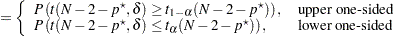### Analyses in the ONECORR Statement

#### Fisher’s z Test for Pearson Correlation (TEST=PEARSON DIST=FISHERZ)

Fisher’stransformation (Fisher 1921) of the sample correlationis defined as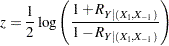Fisher’stest assumes the approximate normal distribution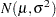for, where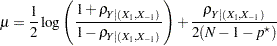and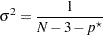where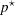is the number of variables partialed out (Anderson 1984, pp. 132–133) andis the partial correlation between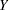and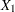adjusting for the set of zero or more variables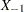.

The test statistic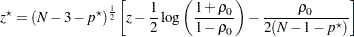is assumed to have a normal distribution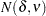, where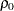is the null partial correlation and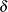and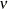are derived from Section 16.33 of Stuart and Ord (1994):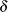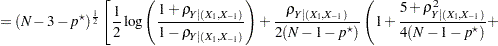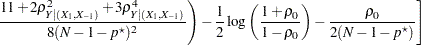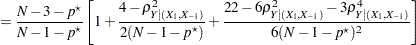The approximate power is computed as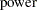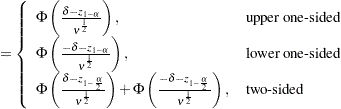Because the test is biased, the achieved significance level might differ from the nominal significance level. The actual alpha is computed in the same way as the power except with the correlationreplaced by the null correlation.

#### t Test for Pearson Correlation (TEST=PEARSON DIST=T)

The two-sided case is identical to multiple regression with an intercept and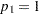, which is discussed in the section Analyses in the MULTREG Statement.

Letdenote the number of variables partialed out. For the one-sided cases, the test statistic is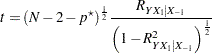which is assumed to have a null distribution of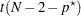.

If the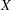andvariables are assumed to have a joint multivariate normal distribution, then the exact power is given by the following formula: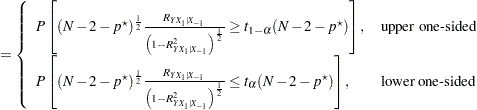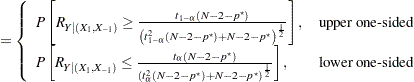The distribution of(given the underlying true correlation) is given in Chapter 32 of Johnson, Kotz, and Balakrishnan (1995).

If thevariables are assumed to have fixed values, then the exact power is given by the noncentraldistribution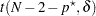, where the noncentrality is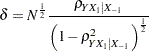The power is